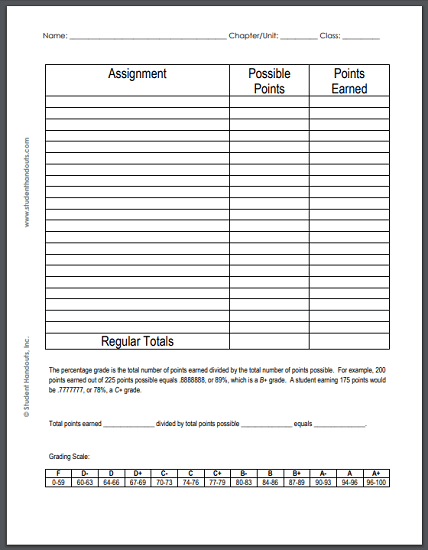﻿
How to Calculate Student Grades | Student Handouts
 There is no set formula for how a teacher must calculate grades.  The only true requirements are that the grading system be fair and consistent.  It is also useful to use a system that allows everyone (parents, students, and teachers) to know a student's current course grade quickly and easily.  One easy way to do this is to assign chapter (or unit) grades.  For example, there are 24 chapters in a textbook, and the teacher issues a test for each chapter.  This amounts to 12 chapters per semester, or 6 chapters per quarter.  (The number of chapters per quarter or semester is actually irrelevant.)  For each chapter (or unit), the teacher assigns point values to various assignments.
 For example: Homework #1 - 10 points Homework #2 - 10 points Homework #3 - 15 points Homework #4 - 15 points Homework #5 - 15 points Group Work #1 -  15 points Group Work #2 - 15 points Participation - 10 points Writing Exercise - 20 points Test - 100 points The chapter (or unit) above totals 225 possible points.  If a student earns 200 out of these 225 points, 200 is divided by 225 for a percentage chapter/unit grade of 89%.
 Some teachers may decide to create a sheet with a table listing each assignment, its point value, the points earned by the student, and the final percentage grade earned by the student.  These sheets are easy to create, and the only writing required on each individual sheet is the student's name and scores.  Curving grades is as simple as changing the total number of points possible to the total points earned by the highest-scoring student.